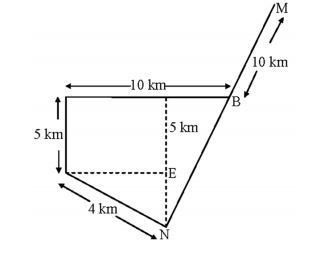Home » Verbal Ability » Numerical Ability » Question

#### Numerical Ability

1. M and N start from the same location. M travels 10 km East and then 10 km North-East. N travels 5 km South and then 4 km South-East. What is the shortest distance (in km) between M and N at the end of their travel.
1. 18.60
2. 22.50
3. 20.61
4. 25.00
##### Correct Option: C

From the given data, the following diagram is possiblecos 45° = DE 4

DE = cos45° × 4 = 2.828 km
 sin 45° = EN 4

EN = sin 45° × 4 = 2.828 km
 sin 45° = EN 4

EN = sin 45° × 4 = 2.828 km
CN = NE + CE = 2.828 + 5 = 7.828 km
CB = AB – AC = 10 – 2.828 = 7.171 km
(NB)² = (NC)² + (BC)²
= (7.828)² + (7.171)²
∴ NB = √(7.828)² + (7.171)²
= 10.616 km
∴ NM = NB + BM = 10.616 + 10
= 20.61 km